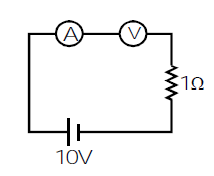Engineering Jobs   »   electrical gate quiz

# GATE’22 EE: Daily Practices Quiz 21-July-2021

Q1-Q2 Carry 1 mark each
Negative marking: 0.33 mark

Q3-Q5 carry 2 marks each
Negative marking: 0.66 mark

Total Questions: 05
Total marks: 8
Time: 12 min.

Q1. The Charging reactance of 50 km length of line is 1500 Ω. What will be the charging reactance for 100 km length of the line?
(a) 600 Ω
(b) 3000 Ω
(c) 750 Ω
(d) 1500 Ω

Q2. Turn off time of an SCR is measured from the instant:
(a) Anode current becomes zero
(b) Anode voltage becomes zero
(c) Gate current becomes zero
(d) Anode voltage and current become zero at the same time

Q3. A 3 phase, 50 Hz induction motor draws 50 kW from the mains. If the stator losses are 2 kW and rotor emf oscillates at 100 oscillations per minute the rotor copper losses will be:
(a) 1.8 kW
(b) 1.2 kW
(c) 1.9 kW
(d) 1.6 kW

Q4. Consider the following statements S_1 and S_2 for the circuit shown in figure below.S_1: the voltage source is delivering 400 W of average power.
S_2: the voltage source is absorbing 200 VAR of reactive power.
Which of the above statements are true?
(a) S_1 only
(b) S_2 only
(c) Both S_1 and S_2 only
(d) Neither S_1 nor S_2

Q5. In the shown figure1. If both ammeter and voltmeter are ideal, then reading of ammeter is zero, reading of voltmeter is 10 V
2. If both ammeter and voltmeter are ideal, then reading of ammeter is 10 A, reading of voltmeter is 0 V
3. If ammeter is non-ideal, voltmeter is ideal, then reading of ammeter is 0 A, reading of voltmeter is 10 V
4. If ammeter is ideal, voltmeter is non-ideal, then reading of ammeter is less than 10 A, reading of voltmeter is less than 10 V
Which of these statements are correct?
(a) 1,2 and 3 only
(b) 2,3 and 4 only
(c) 1, 3 and 4 only
(d) All of the above

SOLUTIONS
S1. Ans.(c)
Sol. charging reactnce(X_C ) α 1/L
∴X_C2/X_C1 =L_1/L_2
⇒X_C2=1500×50/100= 750 Ω

S2. Ans.(a)
Sol. Turn off time of an SCR is measured from the instant when anode current becomes zero.

S3. Ans.(d)
Sol. Slip (s)= f_r/f_s =(100/60)/50
= 1/30
Rotor input = stator input – stator loss
= 50-2 = 48 kw
Rotor copper loss = S × Rotor input
= 1/30×48
= 1.6 kw

S4. Ans.(c)
Sol.Total impedance, Z = 10 + [-j10||(10+j10)]
=10+(-j10)(10+j10)/(-j10+10+j10)
=10+(-j10)(10+j10)/10
= 10+[-j10+10]=20-j10Ω
I_rms=(100∠0° )/Z=100/(20-j10)=4+j2A
Complex power absorbed by the source
S= -V_rms I_rms^* (Passive sign convention)
S = -100(4 – j2) = -400 + j200 = P + jQ
Real power, P = -400 W (absorbed)
Or P = 400 W (delivered)
Reactive power, Q = 200 VAR (absorbed
Both S_1 and S_2 are true.

S5. Ans.(c)
Sol.
If both are ideal, voltmeter has infinite resistance and hence, no current flows through the circuit. Thus, ammeter will read zero and voltmeter will read 10V.
If ammeter is non-ideal but voltmeter is ideal, current through circuit will be 0 and hence ammeter will read 0A and voltmeter 10V.
If voltmeter is non-ideal, finite current will flow through the circuit and hence there will be some voltage drop across the resistor. Therefore, Voltmeter will read less than 10V. Due to finite resistance of voltmeter, current will be less than 10A and hence ammeter will read less than 10A.

Sharing is caring!